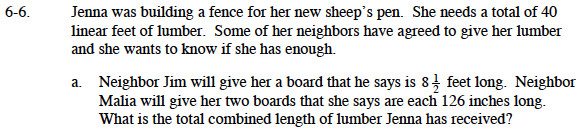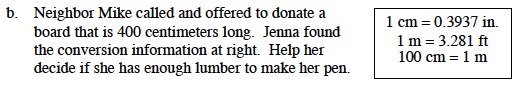### Home > MC1 > Chapter 6 > Lesson 6.1.1 > Problem6-6

6-6.The best way to determine what length Jenna has is to put all the board lengths into the same units. Using feet will make it easier to determine if Jenna has enough at the end. Jim has 8.5 feet to give. Malia has 2(126) inches. How many feet is 2(126) inches?

Can you do this calculation in your head? There are 12 inches in 1 foot, therefore 120 inches is 10 feet.100 cm = 1 m
Therefore, 400 centimeters is equal to 4 meters.
How many feet is 4 meters?